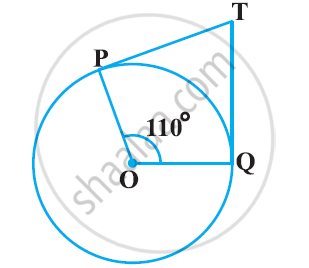# In the given figure, if TP and TQ are the two tangents to a circle with centre O so that ∠POQ = 110°, then ∠PTQ is equal to - Mathematics

MCQ

In the given figure, if TP and TQ are the two tangents to a circle with centre O so that ∠POQ = 110°, then ∠PTQ is equal to• 60°

• 70°

• 80°

• 90°

#### Solution

It is given that TP and TQ are tangents.

Therefore, radius drawn to these tangents will be perpendicular to the tangents.

Thus, OP ⊥ TP and OQ ⊥ TQ

∠OPT = 90º

∠OQT = 90º

Sum of all interior angles = 360°

∠OPT + ∠POQ +∠OQT + ∠PTQ = 360°

⇒ 90°+ 110º + 90° +∠PTQ = 360°

⇒ ∠PTQ = 70°

Hence, alternative 70° is correct.

Concept: Number of Tangents from a Point on a Circle
Is there an error in this question or solution?
Chapter 10: Circles - Exercise 10.2 [Page 213]

#### APPEARS IN

NCERT Class 10 Maths
Chapter 10 Circles
Exercise 10.2 | Q 2 | Page 213

Share